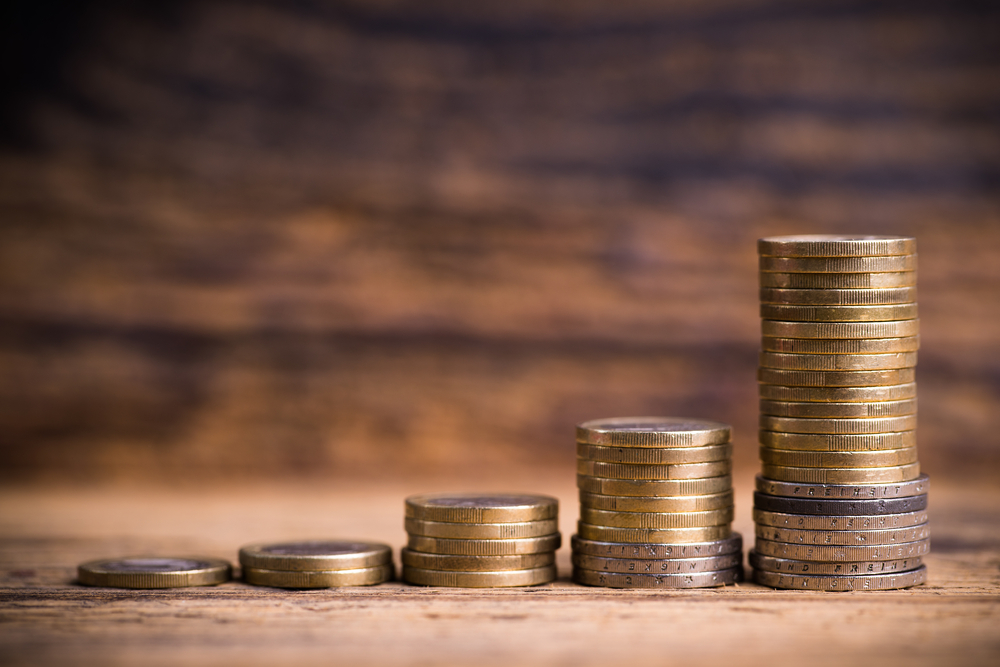# The Magic of Compounding Interest

### What Compound Interest IsCompound interest is the idea of interest on interest, and it is a crucial concept to understand in stocks and savings. Essentially, compound interest means that the sum of interest and principal amounts are added together at the end of a compounding period, and then further interest grows off of that. This differs from simple interest, where the interest is only calculated from the principal amount of a loan or investment. For this reason, a lower compound interest the compounds with short compounding periods will often add up to a higher amount than a high interest rate that is merely simple interest.

### Compounding Period

The frequency that interest is compounded depends on the terms of a specific agreement, whether it be a loan that must be paid back, or the amount that grows on an investment. For example, the compounding period at a bank is daily, which means that interest accrues each day. For most loans, the compounding period is monthly. When it comes to investments, interest is more likely to compound annually, or even semi-annually.

### The Concept of Money’s Time Value

The time value of money explains how an investment can accomplish exponential growth, thanks to compound interest. Essentially, this concept is about how money today is worth more than the same amount of money later in time, because you can spend, utilize, and invest the money that you have today (that’s before considering that inflation lowers the value of money, over time). By considering the time value of money, you can determine the future value of money, and what you’d want your investments to reach in value over a determined period of time.

### The Rule of 72

When considering the time value of money, one common equation is called the “rule of 72”. The rule of 27 helps investors determine how long it will take an investment to double, based on return on compounding interest. The rule of 72 only works for interest that compounds annually. The equation is quite simple. Essentially, if interest is “i”, then you simply divide 72 by the interest amount. For example, an investment with a returning interest rate of 12% will double within 6 years.

### Get Financial Planning Advice, Today!

If you or someone you know needs financial advice or wants to learn more about investments, estate planning, or insurance, then don’t hesitate to give the team at Afortus financial a call, today!